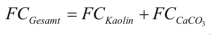# How is the paper density measured

## 2.4.1.2 Basic properties

The basic properties of paper, cardboard and cardboard are the weight per unit area, the thickness, the apparent sheet density, the dry content and the residue on ignition (ash content).
The determination of the area-related mass is carried out in accordance with DIN EN ISO 536.
The basic property is defined as the mass of a unit area of ​​paper or cardboard. It is given in g / m². The measurement is carried out as follows: At least 20 samples with> 500 cm² from at least five test pieces are weighed. You therefore need an analytical balance and tools for cutting samples and for precisely measuring the sample surfaces. Specially designed circular sample punches with a diameter of D = 11.285 cm such that the punched samples have an area of ​​A = 100 cm² have proven to be useful.

The procedure for the determination is explained in Figure 97.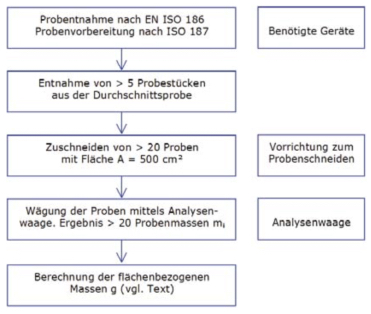Fig. 97: Procedure for determining the area-related mass (source: own illustration)

The mass per unit area g of each sample is to be calculated according to:If the mass is determined in g and the area in cm², then the mass per unit area results in the unit g / m².

Note: Even if the standard uses the symbol g for the mass per unit area, in practice the symbol m has been usedA. enforced. The calculation formula is: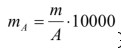To calculate the average area-related mass, the mean value is first used m of the sample masses determined from the i individual values: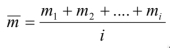The mean value is analogous Ā to determine the sample areas. This can generally be avoided if the above-mentioned sample punch is used to produce the samples, because then all samples have the same area A = 100 cm².

Then proceed as follows to calculate g: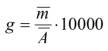Note: Another advantage of the sample punch is that the samples produced with it with A = 100 cm² make evaluation particularly easy. In this case, the value read on the analytical balance in g multiplied by 100 is equal to the mass per unit area in g / m². For example, if a value of 0.85 g is read on the analytical balance, the mass per unit area is 85 g / m².

The second basic property is that thickness, for the measurement of which a special measuring instrument (micrometer) is required, the properties of which are specified in DIN EN ISO 534. It essentially consists of a fixed and a movable punch, the ground end faces of which are exactly parallel to each other. The thickness of a paper sample lying between the stamps is defined by the distance between the two stamps when they act on the paper under the following conditions:

• Stamp area: 200 mm²
• Surface pressure: 100 ± 10kPa
• Lowering speed of the moving ram: <3 mm / s
• Error in plane parallelism: <5 μm

The method of thickness measurement is specified in the above-mentioned DIN EN ISO 534. The procedure is illustrated in Figure 98.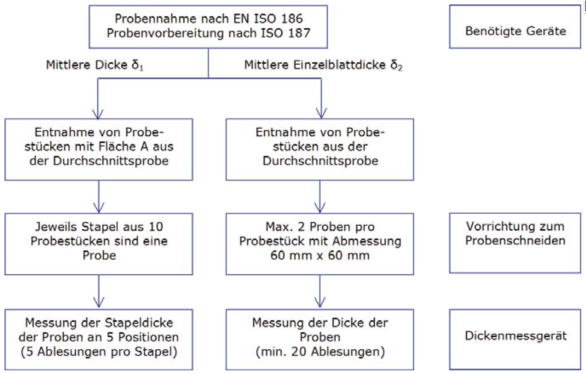Fig. 98: Procedure for measuring the average thickness (measured on the stack) and the average individual sheet thickness. For the evaluation of the measured value readings see text (source: own illustration)

The mean thickness δ₁ is made by adding the five measured value readings from at least four stacks (at least 5 x 4 = 20 readings) and dividing the result by the number of readings. The mean value determined in this way still has to be divided by the number of test pieces in the stack - preferably 10.

At the mean single sheet thickness δ₂ the at least 20 measured value readings are added; the result is divided by the number of readings.

The same standard also specifies how that apparent density ρ as the apparent stacking density ρ? preferably for paper or as an apparent sheet density δ? is to be determined preferably for cardboard and cardboard. In contrast to the mass per unit area g and the thickness δ, it is not measured, but calculated from these quantities - namely, the following applies to the apparent stacking density ρ? with the symbols for the mass per unit area g according to the standard or mＡ according to practice: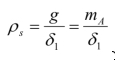And for the apparent sheet density δ ?: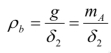If the mass per unit area is given in g / m² and the thickness in μm, the apparent density results in the unit g / cm³. The reciprocal of the apparent density is the specific volume, given in the unit cm³ / g.

The term "apparent density" is based on the fact that the measurement of the thickness, which is needed to calculate the apparent density, is problematic for epistemological reasons. The calculation of the density is based on a measured value, of which one does not exactly know whether it is "correct". This is what gives the name "apparent density". Colloquially, instead of “apparent density”, “raw density” is often used. However, this term does not conform to the standard. Nor does he refer to the problem of thickness measurement.

This is very important for all paper fabrics Moisture content or the Dryness.
Due to its hygroscopic character, paper has certain moisture contents depending on its composition and the ambient climate. The moisture content can differ from place to place, so that a longitudinal and transverse profile can be established in a paper web. Since many paper properties are dependent on the moisture content, there is a corresponding longitudinal and transverse profile of these properties.
The test standard for the moisture content is DIN EN 20287. A drying cabinet, an analytical balance and a desiccator are required to determine the moisture content.
A desiccator is a container, usually made of glass, closed with a lid, in which extremely dry air can be generated using a desiccant. To fill the desiccator with the sample and to remove it, the lid may only be removed briefly.
The procedure for determining the moisture content or the dry content is explained in a simplified manner in Figure 99. When the values ​​are determined in accordance with the standards, the particularities of sampling and sample preparation in DIN EN 20287 must be observed.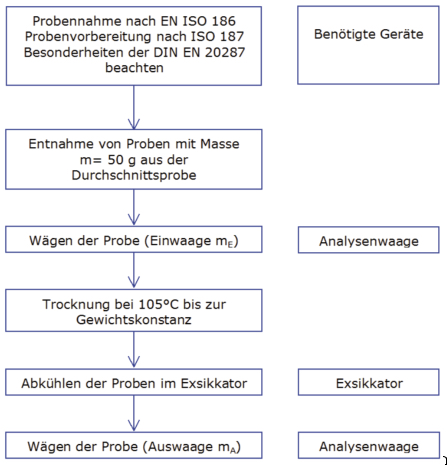Fig. 99: Simplified procedure for determining the moisture or dryness content. The evaluation is explained in the text (source: own illustration)

The moisture content is now calculated in% from the initial weight mE and the final weight mＡ. DIN EN 20187 does not provide for a formula symbol for the moisture content. That is why the frequently encountered FG is used here as a symbol: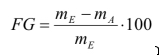The dry content, which is closely related to the moisture content, is determined in accordance with DIN EN 20638, which, more precisely, is intended for pulp testing. In contrast to the determination of the moisture content, the initial weight in my opinion is only about 10 g. The symbol X is used in the standard for the dry content. In practice, however, TG is written more often instead. The dry content TG in% is then: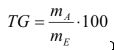Finally, that counts Ignition residue (often also referred to as ash content) on the basic properties of paper. This is understood to be the amount of residue that remains when a sample is burned at a specified temperature. The non-combustible inorganic constituents of paper are recorded - such as the fillers or their residues after burning at high temperatures.

The test standard is DIN 54370. In method A, it provides an annealing temperature of 575 ° C ± 25 ° C and in method B an annealing temperature of 900 ° C ± 25 ° C. The glow time is about three hours. The amount of sample material (initial weight) depends on the expected amount of ignition residue. It is between 50 g for ignition residues up to 0.04% and 5 g for ignition residues above 0.5%.

An annealing furnace and sample crucibles made of ceramic or porcelain, an aluminum block for cooling and a desiccator are required for the determination. The procedure is explained in Figure 100.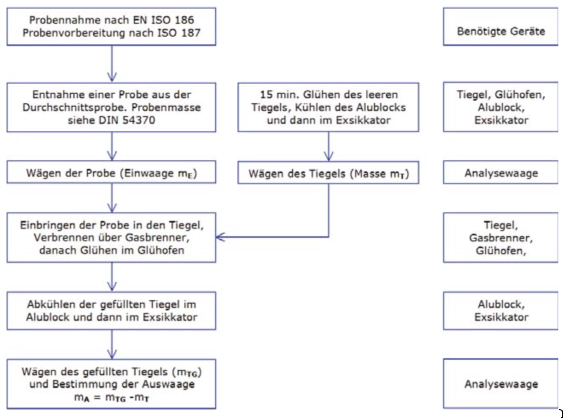Fig. 100: Procedure for determining the residue on ignition. The evaluation of the weighing results is explained in the text (source: own illustration)

From the mass of the paper sample mE (initial weight), the mass of the residue on ignition mＡ (weight) and the dry content TG of the sample, the residue on ignition GR in% results as follows: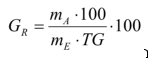Caution is advised if conclusions regarding the filler content FC are to be made from the ignition residue GR according to method B (900 ° C). A frequently used filler is calcium carbonate (CaCO₃). At temperatures above 800 ° C, CaCO₃ decomposes, releasing carbon dioxide (CO₂) into calcium oxide (CaO). Since CaO is lighter than CaCO₃, the residue on ignition does not match the filler content FC, rather GR> FCCaCO₃. A second annealing test according to method A (575 ° C) must then be carried out. The difference between the ignition residues multiplied by the correction factor 2.29 then indicates the CaCO₃ content in%: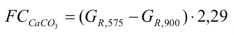If kaolin is used as a further filler in addition to CaCO₃, which does not disintegrate at 900 ° C, the kaolin content of FCKaolin is determined according to the following relationship: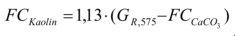The total filler content of the sample is - if no other filler types were used: